Next: Motion in Central Field Up: Orbital Angular Momentum Previous: Rotation Operators

# Eigenfunctions of Orbital Angular Momentum

In Cartesian coordinates, the three components of orbital angular momentum can be written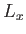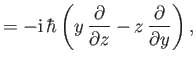(4.74)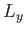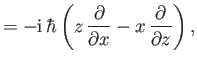(4.75)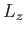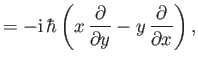(4.76)

using the Schrödinger representation. Transforming to standard spherical coordinates,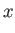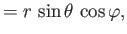(4.77)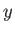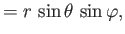(4.78)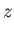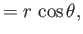(4.79)

we obtain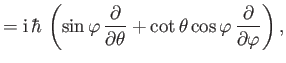(4.80)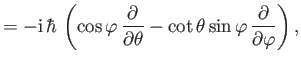(4.81)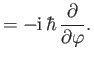(4.82)

(See Exercise 2.) Note that Equation (4.82) accords with Equation (4.57). The ladder operators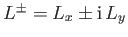become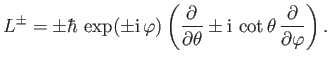(4.83)

(See Exercise 2.) Now,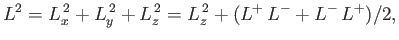(4.84)

so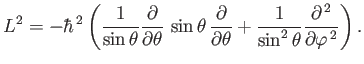(4.85)

(See Exercise 2.)

The eigenvalue problem for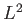takes the form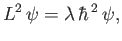(4.86)

where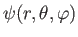is the wavefunction, and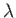is a dimensionless number. Let us write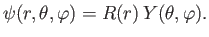(4.87)

Equation (4.86) reduces to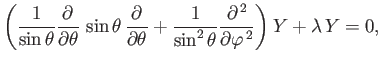(4.88)

where use has been made of Equation (4.85). As is well known, square-integrable solutions to this equation only exist whentakes the values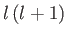, whereis an integer (which we can take to be non-negative, without loss of generality) . These solutions are known as spherical harmonics , and can be written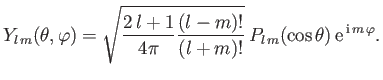(4.89)

Here,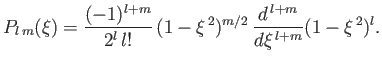(4.90)

is an associated Legendre function , satisfying the equation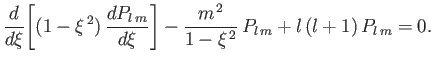(4.91)

It follows that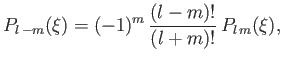(4.92)

and, hence, that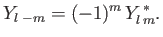(4.93)

Of course,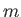must be an integer, so as to ensure that the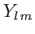are single valued in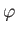. Moreover, it is clear from Equations (4.90) and (4.92) that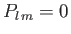unless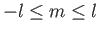. The spherical harmonics are orthogonal functions, and are properly normalized with respect to integration over the entire solid angle :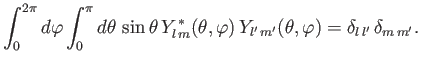(4.94)

The spherical harmonics also form a complete set for representing general functions of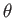and. The first few spherical harmonics are :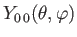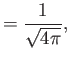(4.95)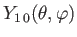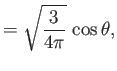(4.96)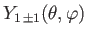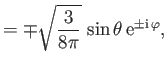(4.97)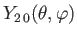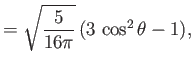(4.98)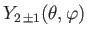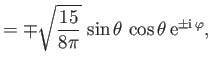(4.99)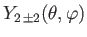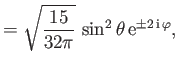(4.100)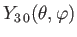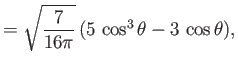(4.101)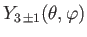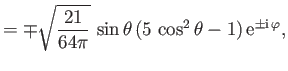(4.102)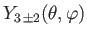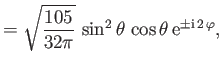(4.103)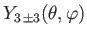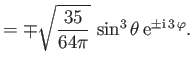(4.104)

By definition,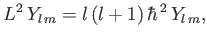(4.105)

whereis an integer. It follows from Equations (4.82) and (4.89) that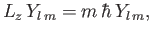(4.106)

whereis an integer lying in the range. Thus, the wavefunction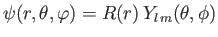, where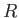is a general function, has all of the expected features of the wavefunction of a simultaneous eigenstate ofand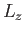belonging to the quantum numbersand. The well-known formula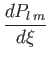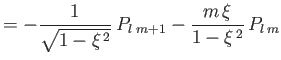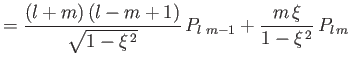(4.107)

can be combined with Equations (4.83) and (4.89) to give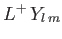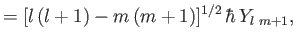(4.108)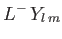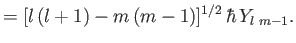(4.109)

(See Exercise 4.) These equations are equivalent to Equations (4.55)-(4.56). Note that a spherical harmonic wavefunction is symmetric about the-axis (i.e., independent of) whenever, and is spherically symmetric whenever(because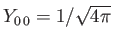).

In summary, by solving directly for the eigenfunctions ofandin the Schrödinger representation, we have been able to reproduce all of the results of Section 4.2. Nevertheless, the results of Section 4.2 are more general than those obtained in this section, because they still apply when the quantum numbertakes on half-integer values.Next: Motion in Central Field Up: Orbital Angular Momentum Previous: Rotation Operators
Richard Fitzpatrick 2016-01-22